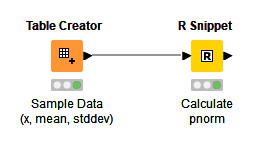# Normal Distribution Density Function (NORM.S.DIST in Excel)

Hi,

I’d like to calculate the probability of an event using the terms I have to normally use NORM.S.DIST in excel (x, mean and standard deviation).

Do you guys have any tips how can I apply it to multiple rows in Knime?

Thank you a lot,

Leo

I think you’re doing to need to give more details here. From your post it’s unclear what exactly it is you’re trying to accomplish.

Hi, elsamuel,

I need to calculate the density of a normal distribution, in which I know the x, mean and standard deviation o its’ formula.

I’d appreciate if you could help me knowing more now.

Thanks,

Leo

I don’t believe there’s a node that will calculate this natively, but the `NORM.DIST` function in Excel is equivalent to the `pnorm` function in R. This means we can use an R Snippet node to do the calculation. It ends up being 3 lines of code, 2 of which are just to move the data into and out of R. Here’s an example:

I just used a small table with 3 rows of made-up input data, but of course you you can use something more meaningful:Give it a try and see what you think.

2 Likes

This topic was automatically closed 182 days after the last reply. New replies are no longer allowed.